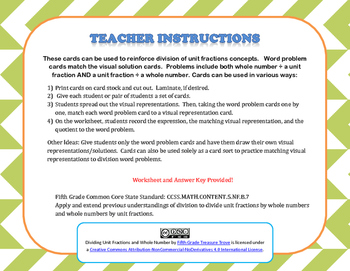# Dividing Unit Fractions and Whole NumbersSubject
Resource Type
File Type
PDF (909 KB|10 pages)
Standards
\$2.00
• Product Description
• Standards
Reinforcing the use of visual representations to solve fraction division problems is easy with these task cards! Students gain practice not only analyzing word problems, but matching the visual solution to each, which aids in true understanding!

This set includes twelve word problem cards with matching visual representation cards. The problems include division of unit fractions by whole numbers and division of whole numbers by unit fractions. A worksheet is included for students to record the expression, matching visual representation, and quotient for each card. Can be printed in grayscale.

Check out some of my other task cards and games!
Fraction Word Problems
Fraction Multiplication 4 in a Row
Apply and extend previous understandings of division to divide unit fractions by whole numbers and whole numbers by unit fractions.
Total Pages
10 pages